American Journal of Modern Physics
Volume 5, Issue 3, May 2016, Pages: 30-38

Review Article

Effect of Pressure and Hot Filament Cathode on Some Plasma Parameters of Hollow Anode Argon Glow Discharge Plasma

Sh. Kh. Al-Hakary

Department of Physics, Faculty of Sciences, University of Zakho, Duhok, Iraq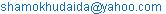Sh. Kh. Al-Hakary. Effect of Pressure and Hot Filament Cathode on Some Plasma Parameters of Hollow Anode Argon Glow Discharge Plasma. American Journal of Modern Physics. Vol. 5, No. 3, 2016, pp. 30-38. doi: 10.11648/j.ajmp.20160503.12

Received: April 1, 2016; Accepted: April 25, 2016; Published: May 23, 2016

Abstract: The present study represents a review article and a complementary study to a published paper of direct current hot cathode and hollow anode argon glow discharge plasma at different pressures in the range 0.05 - 0.8 mbar. The glow discharge usually obtained by applying a high voltage between the electrodes sufficient to occurring breakdown of argon gas and sustaining the discharge. Additional estimation of some plasma parameters, ion temperature, degree of ionization and electron energy distribution function as well as some characteristics of glow discharge under the influence of pressure and filament current has been done. Plasma parameters have been calculated using single probe method at fixed discharge current Id=1.88mA and diameter of hollow anode. Furthermore a computer MATLAB program is applied for this purpose. Results show that the average discharge voltage increases while its average discharge current decreases according to the increasing of the filament current. On the Contrary the average discharge voltage decrease nearly exponentially whiles its average discharge current increases according to increasing of the gas pressure. Degree of ionization starts to increase from low pressure to medium and then tends to decrease toward high pressure investigated. However it starts to increase to maximum value at filament current 0.9A then tends to decrease at higher current and low pressure. While for high pressures there is no significance change. Ion temperature increases slowly with filament current up to 0.9A and then sharply increases to maximum value at 1.5A. But it decreases for pressures in the range 0.05 - 0.09 mbar and then increases with gas pressure. Electron energy distribution function (EEDFs) decrease according to increases filament current, however, it starts to increase from low pressure to maximum value at medium pressure then tends to increase according to further increases of pressure.

Keywords: Hot Cathode, Hollow Anode, Argon Glow Discharge, Single Probe, Plasma Parameters, MATLAB Program

Contents

1. Introduction

Hot cathode discharge plasma with negative ions is widely used in fusion research, development and in modern plasma processing technologies . The detail of a gas discharge by a hot filament cathode has been studied by many researchers , directly heated high temperature cathodes of refractory metals such as tungsten run electric current of more than several tens of amperes. The electric current makes magnetic field around the cathode wire and the magnetic field cause inhomogeneous emission of electrons from the cathode. Very low electron temperatures have been obtained by . A thermionic emission electron has been got by heating a wire filament, accelerating the electrons by high voltage, and ionizing the analyzed molecules . In such a system, the filament emission current and the ionization rate therefore, one needs to control this. , investigate experimentally the influence of hot filament localized behind the cathode on the electrical breakdown characteristics of argon and nitrogen gases. The results show that an increase of filament current causes decrease of breakdown voltage which is more pronounced in lower pressure , study the ways to produce and control the formation of electron energy distribution function (EEDF) in direct current discharge with hot cathode. The direct - current discharge glows in helium and sustained by the electron current emitted from the heated cathode. , designed and constructed a hot cathode plasma source for a 5- centimeter diameter ion beam source. The ion source can be used in ion beam sputter and deposition applications. , study the influence of the cathode heating from external heat source on the APGD parameters. The results show that additional cathode heating can strongly influence the parameters of the cathode region.

The objective of the current study is a review article and an extension to the published paper , to investigate experimentally the effect of filament current and argon gas pressure on some characteristics of glow discharge as well as upon additional plasma parameters, ion temperature, degree of ionization and electron energy distribution functions at a fixed discharge current (Id=1.88mA) and diameter of hollow anode at different working gas pressure.

2. Experimental Equipment

The discharge cell consists of two movable opposite electrodes enclosed in a cylindrical Pyrex glass tube of 5 cm diameter and 25 cm length. Both sides of tube are opened in order to move the electrodes through them to obtain good homogenous discharge and also to a fixed distance112 mm. Two cylindrical plastic rubbers were used to close both sides of the chamber to prevent the leakage of gas as well as to insert the electrodes through them. Hollow anode is made of copper metal of hollow cylindrical shape of 4cm inter diameter and 3.5cm of hollow depth. Cathode filament was manufactured from tungsten of 1.5cm spiral diameter and 5cm length. The Leybold TRIVAC E vacuum pump (dual-stage rotary vane vacuum pump) was used to evacuate the system to an ultimate pressure 0.00986 mbar. Single probe was constructed from tungsten wire of radius 0.1mm and the length of 6mm which is exposed to discharge. This in turn, inserted to the chamber through a glass tube. "Fig. 1", shows the circuit for measuring the current–voltage characteristics of glow discharge as well as of single probe. The experiments were carried out firstly to evacuate the system to base pressure of 0.00986 mbar. A continues dynamic flow of argon gas (of purity 98%) was let in the system through a needle valve to control the argon gas flow at the desired pressure and thermocouple vacuum gauge is used for measuring pressure. Discharge voltage has been supplied to the electrodes system by a DC power supply (3B power supply U21060), which is a variable power supply of range (0-6000) V, sufficient to make breakdown and obtain the glow discharge. Filament was heated using a DC power supply (3B power supply U21060); it is a variable power supply of range of (0-24) V and maximum current 20A. A digital multi-meter (Mastech M9803R True RMS millimeter) ammeter and voltmeter were used to measure the (V-I) discharge characteristics under different gas pressure and filament current. The non-linear protective resistor (lamp) was used to limit the discharge current and avoid the streamer to pass through the chamber to make the spark breakdown . The probe voltage is obtained from (220-50 HZ), AC power supply bias the probe about 100 Volt using isolated transformer as shown in "fig. 1". Also a direct resistance (10 kΩ) is used to convert the voltage to real value of current through MATLAB program. The capacitor (C50 SAMER 29539) was used to reduce the phase difference in (Ip – Vp) characteristic of probe. The x-y recorder (oscilloscope) traces the (Ip – Vp) characteristic of the probes, and the voltage on the probes is recorded on the x-axis, while the current through the probes is recorded on the y-axis, as the voltage drop across the resistance of value (10 KΩ). More details about the equipment and experimental work are reported by .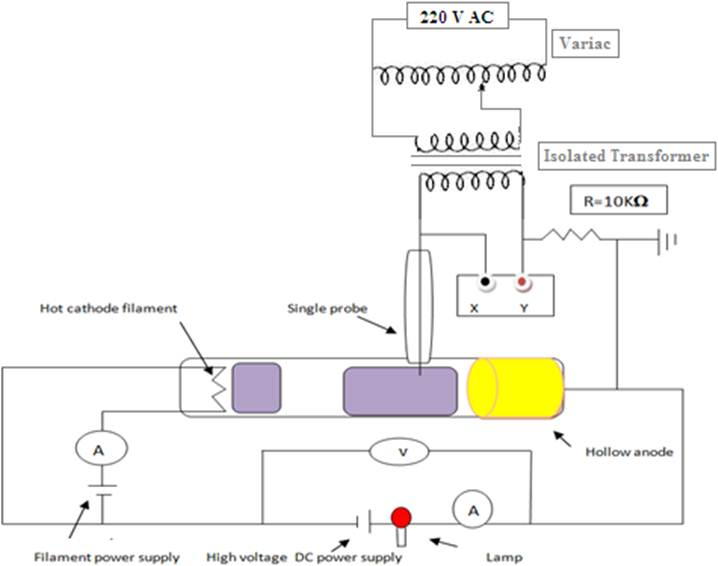Figure 1. Discharge electric circuit.

3. Experimental Results and Discussion

"Fig. 2"and "fig. 3", describe an average of voltage and current versus filament current at pressures 0.03, 0.04 and 0.06 mbar. It appears from the figures that increasing filament current causes increasing of average voltage and decreasing of average current, in other words, discharge resistance increases. The latter, means that increasing emitted electrons from the filament enhances the negative space charge near the anode and consequently the discharge voltage increases as well as its resistance .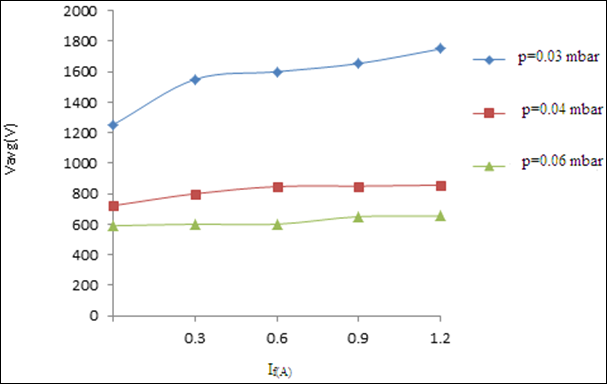Figure 2. Average voltage versus filament current.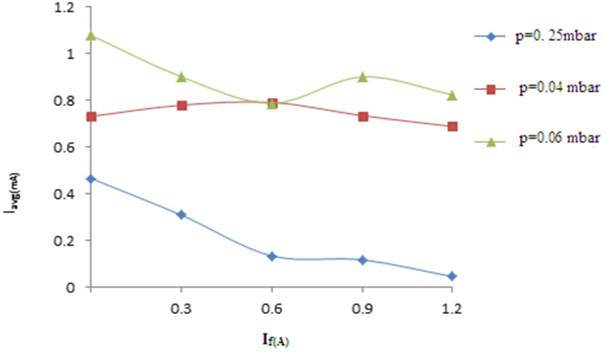Figure 3. Average current versus filament current.

The average voltage and current are plotted as a function of pressure is shown in "fig. 4" and "fig. 5". It is appear from the curve that the average voltage is nearly decreasing exponential with pressure in the range 0.026 - 0.079 mbar. Because this range of pressure represents the left hand branch of Paschenc’s curve and the breakdown versus pressure close to decreasing exponential. It was also observed that average current increases with the increasing pressure.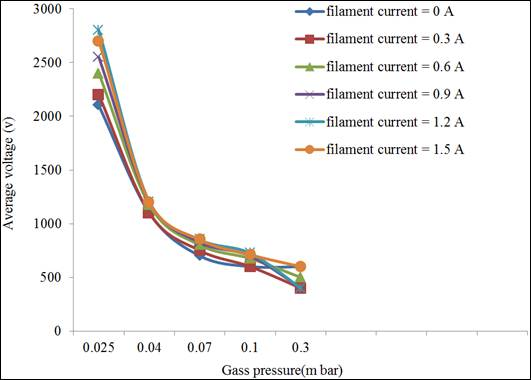Figure 4. Average voltage versus pressure.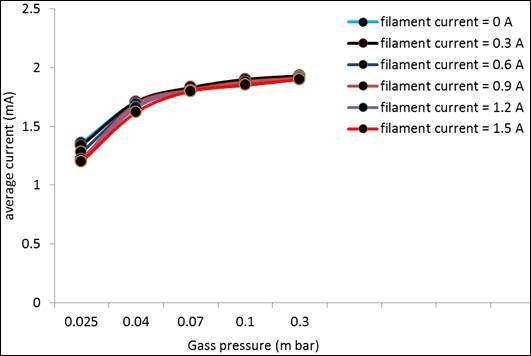Figure 5. Average current versus pressure.

Degree of ionization represents the ratio between the ionized and the neutral particle of the plasma was obtained using the following equation.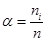(1)

Where ni and n are the density of ionized and neutral particles respectively . "Fig. 6", shows the degree of ionization versus gas pressure at different constant filament current. As shown in the figure the degree of ionization increases from pressure 0.05 to 0.09 mbar and tends to decrease for higher pressure investigated. This is because this range of pressure represents the left hand branch of Paschen’s curve approaching to minimum point (which is represents high α) and towards higher range of pressure that is in the right hand branch of Paschen’s curves .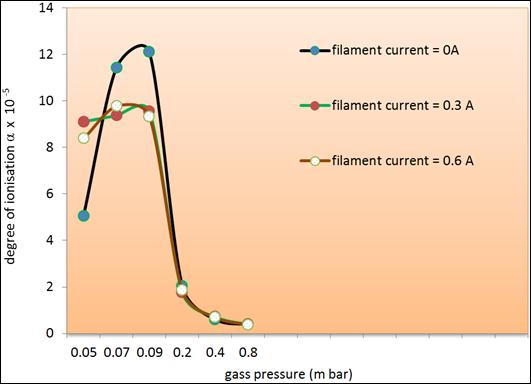Figure 6. Degree of ionization versus gas pressure.

Degree of ionization was plotted as a function of filament current at different constant pressures. "Fig. 7", show that the degree of ionizations according to increasing filament current increases from zero up to 0.9A and then decreases for higher filament current. Since at this range of current, the emitted electron from the cathode contributes to the ionizing collision and consequently the degree of ionizations increases. Furthermore the increasing of filament current thickens the negative space charge near the anode leading to non-ionizing collisions of electron with other plasma particle . This behavior is in well agreement with the previous study . But at high pressures investigated 0.2, 0.4 and 0.8 mbar as shown in "fig. 8". The variation is similar to "fig. 7", for the pressure 0.2mbar, while for the pressures 0.4 and 0.8 mbar, there is no effective variation as shown in figure.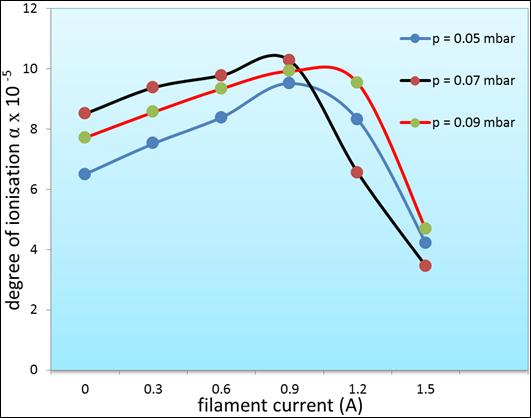Figure 7. Degree of ionization versus filament current.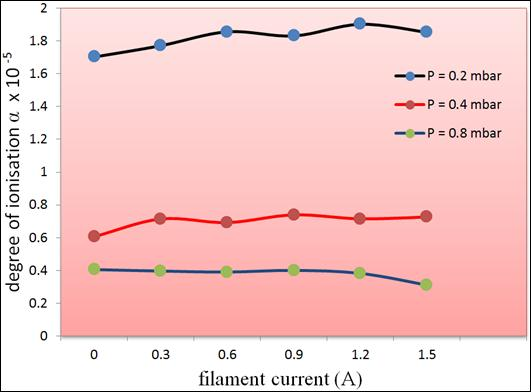Figure 8. Degree of ionization versus filament current.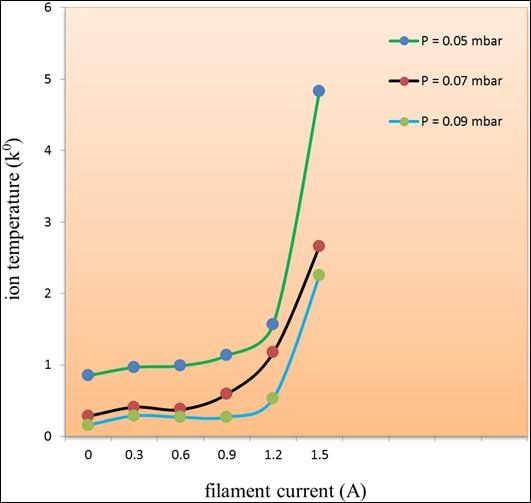Figure 9. Ion temperature versus filament current.

Ion temperature has been calculated using the following equation:(2)

Where Te and Ti are electron and ion temperature of masses me and Mi respectively . "Fig. 9", represents the variation of ion temperature with the filament current and there is no significance increase of ion temperature up to filament current 0.9A due to ionizing collisions of electrons with other particle and increasing electrons density.  Increase of filament current will enhance ion temperature, since latter increasing filament current will increase the negative space charge. Therefore the number will enhance of non-ionizing collisions of electrons with the ions and others plasma particles, and then the ions temperature will increase according to that. Also ion temperature was plotted against the gas pressure as shown in "fig. 10".Figure 10. Ion temperatures against gas pressure.

"Fig. 10", show that the increasing gas pressure from 0.05 to 0.09 mbar causes decreasing ion temperature due to decreasing electron temperature and then tends to increase according to increase of electron temperature . Electron Energy distribution function (EEDFs) is one of the most important property for fusion and processing plasma. As various properties of plasma depends on different part of (EEDFs) such as diffusion coefficient depends on the bulk of the (EEDFs) and ionization or excitation rates depend on the tail of the (EEDFs), the determination of the whole distribution is compelling for a comprehensive characterization of a plasma . Measurements of the (EEDFs) are important in determining the plasma parameters and for optimizing the processes used for various applications . Electron energy distribution function (EEDFs) plays an important role in plasma modeling. Various approaches can be used to describe the (EEDFs), such as Maxwellian, Druyvesteyn, or using a solution of the Boltzmann equation, as well as it is essential in plasma modeling because it is needed to compute reaction rates for electron collision reactions. This is because electron transport properties can also be derived from the (EEDFs) ). In the present study the (EEDFs) are calculated according to both Maxwellian and Druyvesteyn formulas respectively .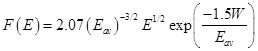(3)(4)

Where Eav and E are the average energy and energy of electron respectively. "Fig. 11", show the electron energy distributions function versus filament current at different gas pressure. The plots indicated that (EEDFs) decrease with the increasing filament current, the amount of high energy electrons decreases. The latter effect is attributed to enhancing the negative space charge near the anode .Figure 11. EEDFs versus filament current.

Later the (EEDFs) are plotted against the gas pressure for different filament current as shown in "fig. 12". It appears from figure there are a few amount of high energy electrons at low pressure and the amount of low energy electrons increase with increasing pressure approaching to 0.09 mbar, then the amount of high energy electrons tends to decease according to further increasing gas pressure, since the electron can transfer almost all its energy to the heavy particle due to inelastic collisions creating an energetic plasma species . Therefore it can be concluded that there are three groups of electrons: group of high energy electrons at low, group of high pressures and group of low energy electrons at medium pressure investigated .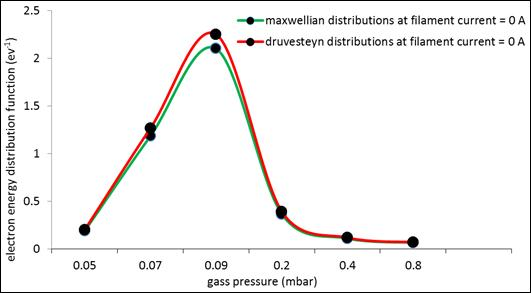Figure 12. EEDFs as a function of pressure.

4. Conclusions

Estimation of some plasma parameters of direct current hot cathode and hollow anode of argon glow discharge plasma at different pressures in the range 0.05 - 0.8 mbar has been carried out. The experiments were conducted under the influence of both pressure and cathode filament current. The most important results are as follows:

1. The average discharge resistance increase according to increase of average voltage and decreases of average discharge current, it decrease nearly exponentially according to increasing gas pressure.

2. Degree of ionization starts to increase from low pressure to medium and then tends to decrease toward high pressure. It is variation according to increasing filament current increases from zero up to 0.9A and then decrease for higher filament current.

3. There is no significance increase of ion temperature up to filament current 0.9A due to ionizing collisions of electrons with other particle and increasing electrons density. Moreover increase of filament current will enhance ion temperature

4. Electron energy distribution function (EEDFs) decrease according to increasing filament current.

5. There are three groups of electrons: group of high energy electrons at low pressure, a group at high pressures and a group of low energy electrons at medium pressure.

6. For low filament current and low pressure the obtained values of (EEDFs) using Druyvesteyn’s distribution is higher than what using Maxwell’s distribution.

7. It can be concluded that the Druyvesteyn’s distribution gives reasonable indication of (EEDFs) at low pressure.

References

1. M. Cercek, T. Gyergyek "Structure of discharge plasma with negative ions model and simulation" 31st EPS Conferences on Plasma Phys. London, 28 june-2 July, ECA Vol. 28G P-4.2282004.
2. N. Miyamoto, N. Hamamoto, S. Imakita, A. G. Mendenilla, and M. Wada "Development of a coaxial hot cathode for magnetized ion source plasma "AIP Conf. Proc. Vol. 1066, 304, 2008.
3. W. Handly and S. Robertson "A Hot- filament with very low electron temperature" Phys, Plasmas, Vol. 16, 010702, 2009.
4. E. Flaxer "Programmable smart electron emission controller for hot filament" Rev. Sci . Instrum. Vol. 82, 025111, 2011.
5. K. Yasserian, A. Fateh Borkhari and D. Dorranian "The breakdown characteristics in the presence of a hot filament for Ar and N2 gases" World Applied Science Journal 16 (6): 755-758, 2012.
6. I. V. Schweigrt, I. D. Kaganovich and V. I Demidov "Active electron energy distribution function control in direct current discharge using auxiliary electrodes" Physics of Plasma 20, 01606, and 2013.
7. M. Salahshoor, A. A. Zavarian, M. Salehi and S. M. J. Ghotbi "Constriction of a hot cathode plasma source for ion beam sputter deposition" Iranian Journal of Surface and Engineering, No. 17, PP. 31-35, 2014.
8. V. I. Arkhipenko, L. V. Simonchik "Effect of cathode heating and positive column contraction on the spatial distributions of parameters in cathode region" 32nd ICPIG, July, 2015, 26-31, Iasi, Romania.
9. W. L. Holtgreven "plasma diagnostic" Ed, Ch. 11. North Holland, Amsterdam, Holland, 1968.
10. K. Al-Hakary, Luqman, Doskyand, Shamil K. Talal "Investigation of cathode and hollow anode of argon glow discharge plasma" Applied physics research. Vol. 6, No. 5, 2014.
11. B. Jomachi and B. Klobes "Franck-Hertz experiment" Advanced Laboratory Course, University Bonn, 2005.
12. Grill "Cold plasma in materials fabrication from fundamentals to applications" Institute of Electrical and Electronic Engineers, Inc, New York, USA, 1993.
13. M. Swarnalatha, C. Sravani, K. R. Gunasekhar, G. K. Muralidhar and S. Mohan "Estimation of density of charge species in a triode discharge system "Vacuum, Vol. 48, No. 10, 845-848, 1997.
14. Esho Q Yuokhana, Luqman M. S. Dosky, Shamoo K Al-Hakary " Plasma parameters in hot cathode glow discharge" J. Duhok. University, Vol. 13, No. 1 (Pure and Eng, Science), Pp . 184-190, 2010 .
15. L. Merlio "Understanding Langmuir probe current –voltage characteristics" Am. J. Phys, Vol. 75, No. 12, 2007.
16. Bijal Vara, C S Dalal, S Karkari, H Kabariya "Langmuir probe diagnostic for local parameter measurement in magnetized plasma using Lab VIEW" Bijal Vara et al Int. Journal of Engineering Research and Applications www.ijera.com ISSN: 2248-9622, Vol. 4, Issue 3 (Version1), March, 2014, pp. 244-247.
17. Tsanko Tsankov, Zhivko kiss, Ovski, Nina, Djermanova, Stanimir, Kolev "Electron energy distribution function in an inductively driven tandem plasma source" Plasma processes and polymers, DoI: 10.1002/ppap.200200092, 2005.
18. Annette Meiners "Electron energy distribution function" CONSOL conferences, 2014.

 Contents 1. 2. 3. 4.
Article ToolsAbstractPDF(2790K)